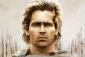# Explain graphically the relation between slope of a tangent line to the function and the derivative.

2 posts / 0 new
John Kervin Triumfo
Explain graphically the relation between slope of a tangent line to the function and the derivative.
Alexanderslope of tangent is the limit of the slope of the secant.

• Mathematics inside the configured delimiters is rendered by MathJax. The default math delimiters are $$...$$ and $...$ for displayed mathematics, and $...$ and $...$ for in-line mathematics.